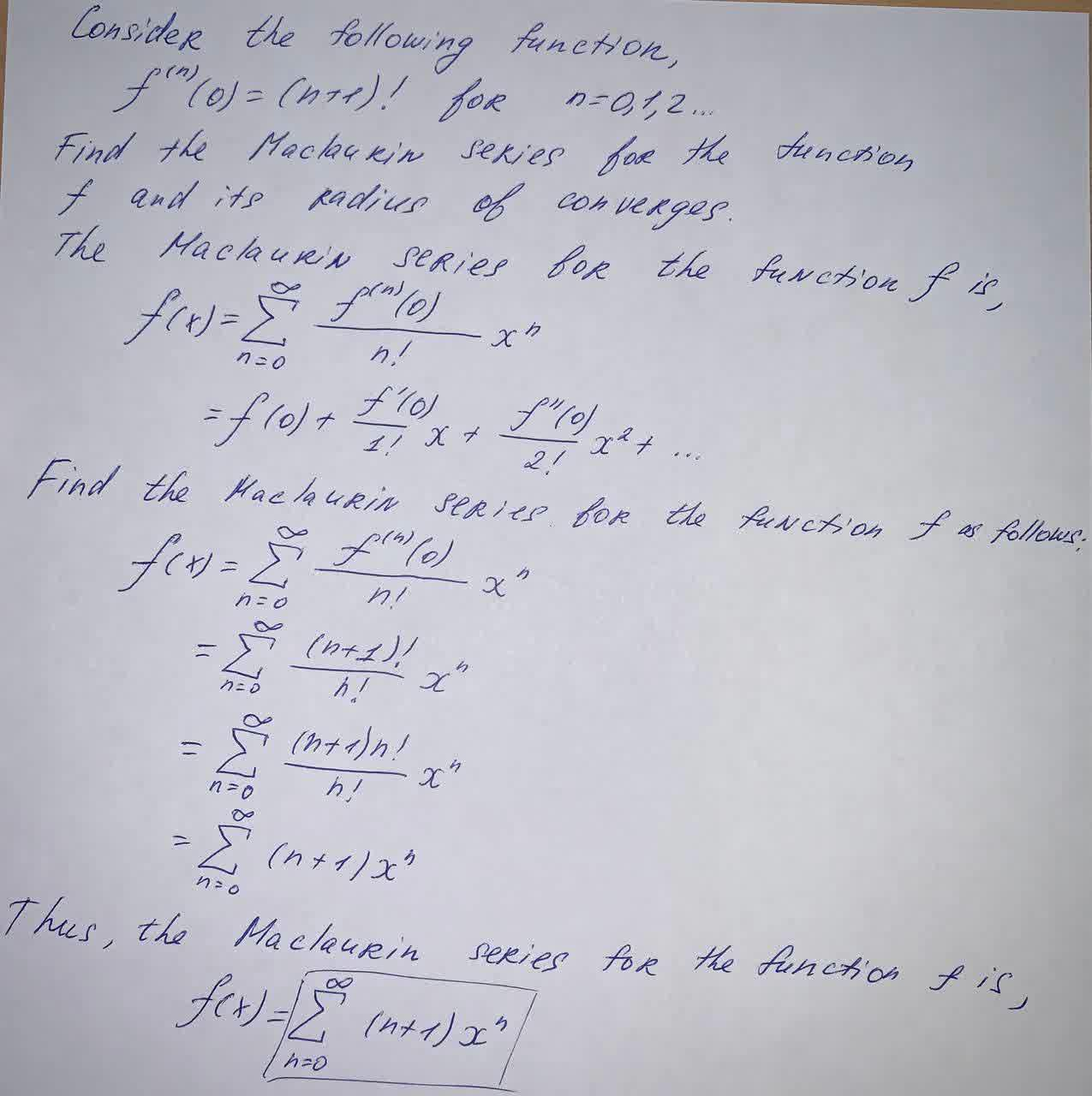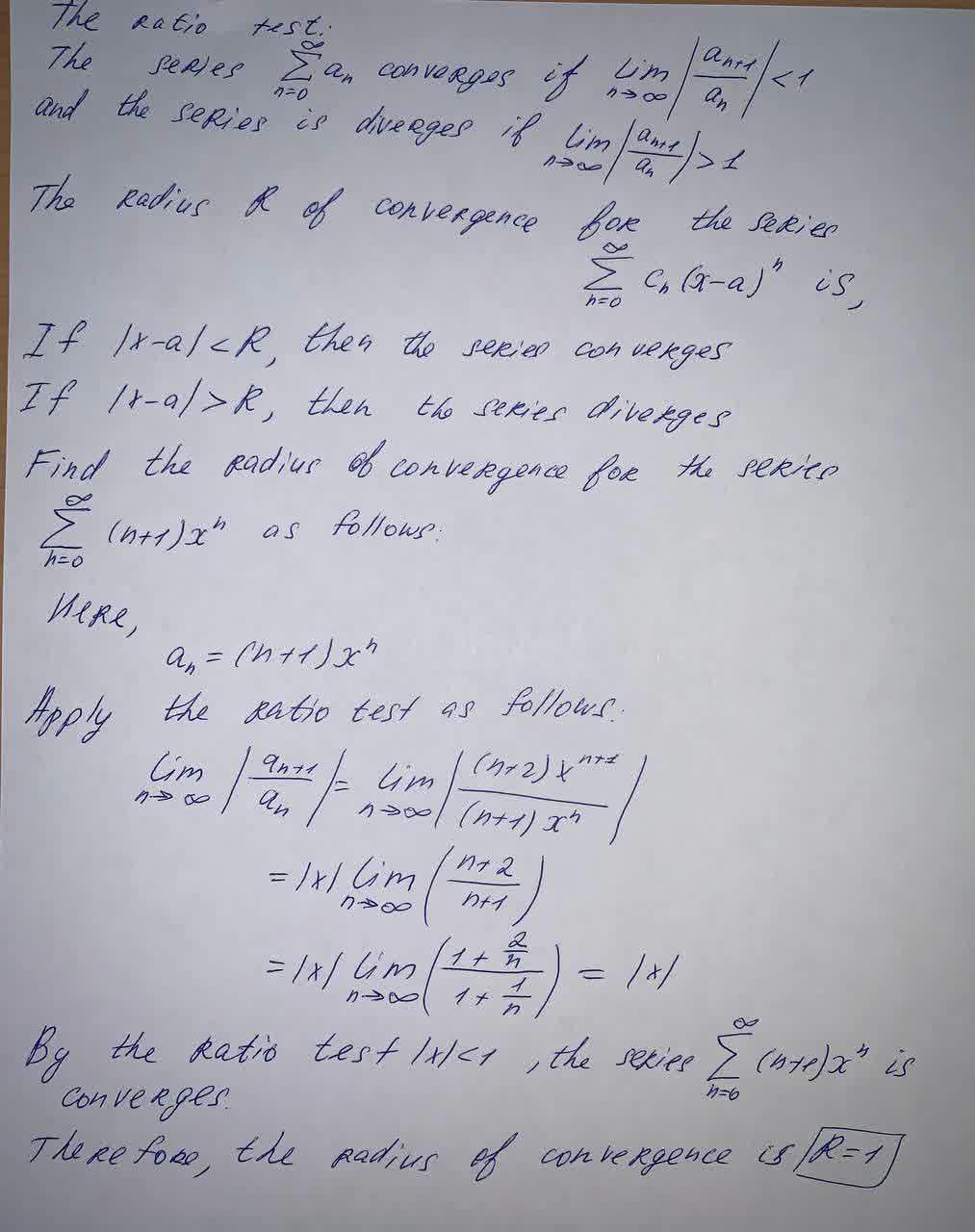# If f^{(n)}(0) = (n+1)! for n = 0, 1, 2, \dots, find the Maclaurin series forf and its radius of convergenceFizeauV 2021-09-05 Answered
If $$\displaystyle{{f}^{{{\left({n}\right)}}}{\left({0}\right)}}={\left({n}+{1}\right)}!\ \text{ for }\ {n}={0},{1},{2},\dot{{s}}$$, find the Maclaurin series forf and its radius of convergence.

• Questions are typically answered in as fast as 30 minutes

### Plainmath recommends

• Get a detailed answer even on the hardest topics.
• Ask an expert for a step-by-step guidance to learn to do it yourself.Demi-Leigh Barrera
At the given condition: